## RS Aggarwal Class 6 Solutions Chapter 5 Fractions Ex 5G

These Solutions are part of RS Aggarwal Solutions Class 6. Here we have given RS Aggarwal Solutions Class 6 Chapter 5 Fractions Ex 5G.

Other Exercises

Objective Questions :
Tick the correct answer in each of the following :

Question 1.
Solution:
(c) ∴ canceling the common factor 2, we get $$\\ \frac { 3 }{ 5 }$$

Question 2.
Solution:
(c) ∴ multiplying numerator and denominator by 4, we get $$\\ \frac { 8 }{ 12 }$$

Question 3.
Solution: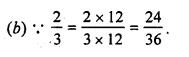Question 4.
Solution: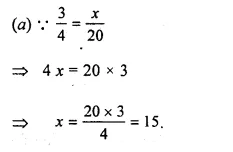Question 5.
Solution: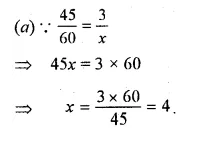Question 6.
Solution:
(c) each of the fractions has the same denominator.

Question 7.
Solution:
(d) none of these has greater denominator than its numerator.

Question 8.
Solution:
(a) its denominator is greater than its numerator.

Question 9.
Solution:
(b) their numerators are same and 4 < 5 , $$\frac { 3 }{ 4 } >\frac { 3 }{ 5 }$$

Question 10.
Solution: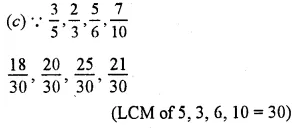Question 11.
Solution:
(b) In $$\frac { 4 }{ 5 } ,\frac { 2 }{ 7 } ,\frac { 4 }{ 9 } ,\frac { 4 }{ 11 }$$ numerator is same then the smallest denominator’s fraction is greater.

Question 12.
Solution:
(a) Denominators are same, then fraction of smallest numerator will be smallest.

Question 13.
Solution: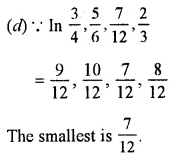Question 14.
Solution:Question 15.
Solution:Question 16.
Solution: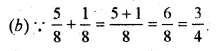Question 17.
Solution: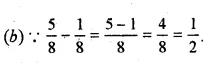Question 18.
Solution: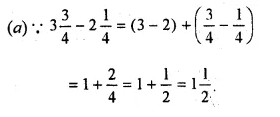Question 19.
Solution: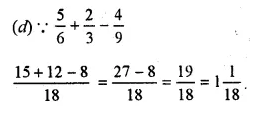Question 20.
Solution: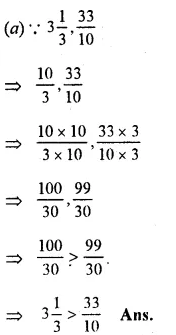Hope given RS Aggarwal Solutions Class 6 Chapter 5 Fractions Ex 5G are helpful to complete your math homework.

If you have any doubts, please comment below. Learn Insta try to provide online math tutoring for you.